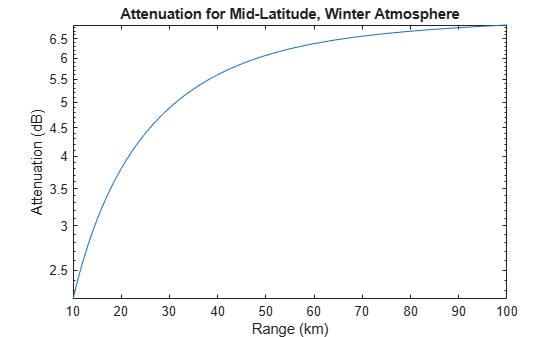# tropopl

Slant-path loss due to atmosphere gaseous absorption

## Syntax

``Lgas = tropopl(R,F,H,EL)``
``Lgas = tropopl(___,Name,Value)``
``[Lgas,Llens] = tropopl(___)``

## Description

````Lgas = tropopl(R,F,H,EL)` calculates the path loss due to tropospheric refraction using the International Telecommunication Union (ITU) standard atmospheric model known as the mean annual global reference atmosphere (MAGRA), which approximates the U.S. Standard Atmosphere 1976 with insignificant relative error.```

example

````Lgas = tropopl(___,Name,Value)` specifies options using one or more name-value arguments in addition to the input arguments in the previous syntax.```
````[Lgas,Llens] = tropopl(___)` calculates the corresponding lens loss. The variation in refractivity versus altitude makes the atmosphere act like a lens with loss independent of frequency. Rays leaving an antenna are refracted in the troposphere and the energy radiated within some angular extent is distributed over a slightly greater angular sector, thereby reducing the energy density relative to propagation in a vacuum.```

## Examples

collapse all

Calculate the attenuation versus range for a frequency of `100` GHz with an elevation of `5` degrees using the mid-latitude, winter atmospheric model.

```R = (10:200)*1e3; % m f = 100e9; % Hz ht = 0; % m el = 5; % deg Lgas = tropopl(R,f,ht,el,'LatitudeModel','Mid','Season','Winter');```

Plot the results.

```semilogy(R.*1e-3,Lgas); xlabel('Range (km)'); ylabel('Attenuation (dB)'); title('Attenuation for Mid-Latitude, Winter Atmosphere');```## Input Arguments

collapse all

Slant range, specified as a positive scalar or an M-length column vector. Units are in meters.

Data Types: `single` | `double`

Radar frequency, specified as a positive real scalar or N-length row vector. Units are in Hz.

Data Types: `single` | `double`

Mean sea level (MSL) altitude of the radar platform, specified as a positive scalar from `0` to `100` km. Values outside the specified range result in `NaN` output. Units are in meters.

Example: `200e3`

Data Types: `single` | `double`

Elevation angle of the propagation path, specified as a scalar. Units are in degrees.

Example: `10`

Data Types: `single` | `double`

### Name-Value Arguments

Specify optional comma-separated pairs of `Name,Value` arguments. `Name` is the argument name and `Value` is the corresponding value. `Name` must appear inside quotes. You can specify several name and value pair arguments in any order as `Name1,Value1,...,NameN,ValueN`.

Example: `'LatitudeModel','Mid','Season','Winter'`

Standard ground-level water vapor density, specified as a positive scalar in `g/m3`. Applicable only for the default standard model (MAGRA). Defaults to 7.5 `g/m3`.

Data Types: `double`

Altitude above mean sea level (MSL), specified as a positive scalar in meters. Applicable only for the default standard model (MAGRA). Defaults to `2e3` meters. For a dry atmospheric conditions, set scale height to `6e3` meters.

Data Types: `double`

Reference latitude model, specified as one of these.

ModelDescription
`'Standard'`(default)

This model is the mean annual global reference atmosphere (MAGRA) that reflects the mean annual temperature and pressure averaged across the world.

`'Low'`

This model is for low latitudes less than `22` degrees, where there is little seasonal variation.

`'Mid'`

This model is for mid latitudes between `22` and `45` degrees with seasonal profiles for `'Summer'` and `'Winter'`, which can be specified using the `'Season'` name-value argument.

`'High'`

This model is for high latitudes greater than `45` degrees with seasonal profiles for `'Summer'` and `'Winter'`, which can be specified using the `'Season'` name-value argument.

Season for the `'Mid'` and `'High'` latitude models, specified as `'Summer'` or `'Winter'`. Other models ignore this input. Defaults to `'Summer'`.

Custom atmospheric measurements for the calculation of the refractive index, specified as an N-by-`4` matrix, where N corresponds to the number of altitude measurements. N must be greater than or equal to 2. The first column is the atmospheric temperature in kelvins, the second column is the atmospheric pressure in hPa, the third column is the water vapor density in g/m3, and the fourth column is the MSL altitude of the measurements in meters. When you use a custom model, all other name-value arguments are ignored and the output refractive index is applicable for the input height.

Note

The model used by `lenspl` assumes geometrical optics conditions, as a result anomalous propagation like ducting and subrefraction cannot be present in provided measurements. If atmospheric measurements evidencing ducting and subrefraction are provided, this function throws an error.

Data Types: `single` | `double`

## Output Arguments

collapse all

Path loss due to tropospheric refraction, specified as an M-by-N matrix. M and N are defined by the slant range, `R`, and frequency, `F`, arguments, respectively. Units are in decibels (dB).

One-way lens loss, specified as an M-by-N matrix for elevation angles less than `50` deg. M and N are defined by the slant range, `R`, and frequency, `F`, arguments, respectively. Units are in decibels (dB).

Data Types: `double`

## Extended Capabilities

### External Websites

Introduced in R2021a